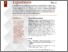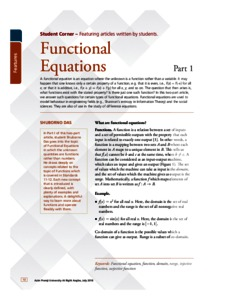# Functional equations

Das, Shuborno (2018) Functional equations. At Right Angles, 7 (2). pp. 10-18. ISSN 2582-1873Preview
Text - Published Version

## Abstract

A functional equation is an equation where the unknown is a function rather than a variable. It may happen that one knows only a certain property of a function; e.g. that it is even, i.e., f (x) = f (–x) for all x; or that it is additive, i.e., f (x + y) = f (x) + f (y) for all x, y; and so on. The question that then arises is, what functions exist with the stated property? Is there just one such function? In this two-part article, we answer such questions for certain types of functional equations. Functional equations are used to model behaviour in engineering fields (e.g., Shannon's entropy in Information Theory) and the social sciences. They are also of use in the study of difference equations.

Item Type: Articles in APF Magazines
Authors: Das, Shuborno
Document Language:
Language
English
Uncontrolled Keywords: Functional equation, function, domain, range, injective function, surjective function
Subjects: Natural Sciences > Mathematics
Divisions: Azim Premji University > University Publications > At Right Angles
Full Text Status: Public
URI: http://publications.azimpremjifoundation.org/id/eprint/1613
Publisher URL: http://apfstatic.s3.ap-south-1.amazonaws.com/s3fs-...View Item